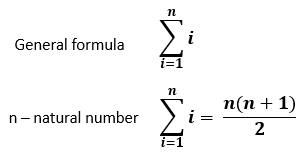# Summation Calculator

Use this summation notation calculator to easily calculate the sum of a set of numbers also known as Sigma, hence this tool is often referred to as a sigma notation calculator. Also outputs a sample of the series to sum. In simple mode it allows the computation of a simple sum given a set of numbers.

Share calculator:

Embed this tool:
get code

## How to calculate a sum?

Summation is the sequential addition on a set of numbers. Addition is one of the four basic arithmetic operations, the others being subtraction, multiplication and division. It is simple to do for a few numbers, especially integers, but can get more complex with fractions and real numbers, which is where our summation calculator can help. You can simply copy/paste the numbers, or enter them manually, separated by any non-numerical symbol - minus and dot excluded. Shortcuts exist for calculating sums of specific sequences.

Since addition is associative, the sum does not depend on how the additions are grouped, therefore, parentheses are usually omitted in summation. Addition is commutative, so permuting (changing the order of) the terms of a finite sequence does not change the result from its summation: summing 1 + 2 + 3 + 4 is the same as 1 + 4 + 3 + 2, as can be confirmed using our sum calculator. Summation can be performed on negative numbers as well, and when one wants to explicitly denote that the sign is taken during the operation, it is called an "algebraic sum".

If you are adding all numbers from a set together, you can refer to the result as "sum total", unlike if you add together only a part of the sequence. A sum of series, a.k.a. summation of sequences is adding up all values in an ordered series, usually expressed in sigma (Σ) notation. A series can be finite or infinite depending on the limit values.

## Using the summation calculator

In "Simple sum" mode our summation calculator will easily calculate the sum of any numbers you input. You can enter a large count of real numbers, positive and negative alike, by separating them using commas, spaces, news lines, tabs, or a combination of the above. Just make sure to use dot (.) as a decimal notation, e.g. 1.5 instead of 1,5 since the latter will be interpreted as two separate numbers, one and five, by our sum calculator.

In "Sigma notation" mode you are to enter the lower and upper limits, a mathematical expression through which each member of the sum series is to be computed, and finally specify what is the name of the variable used in the sigma expression. You can use "pi" and "e" for the number π and the natural logarithm e (a.k.a. the Euler number, ~2.718). It supports all arithmetic operations: + (addition), - (subtraction), * (multiplication), / (division), ^ (raise to power). Parenthesis are used to define groups within the expression.

The sigma notation calculator also supports the following in-built functions, listed alphabetically:

Supported in-built functions for the sigma expression
Function nameAlternative nameWhat it calculates
abs - absolute value
arccos acos arccosine
arccosh acosh hyperbolic arccosine
arcsin asin arcsine
arcsinh asinh hyperbolic arcsine
arctan atan arctangent
arctanh atanh hyperbolic arctangent
cos - cosine
cosh - hyperbolic cosine
ln log natural logarithm
sin - sine
sinh - hyperbolic sine
sqrt square root
tan - tangent
tanh - hyperbolic tangent

In the expression you can enter minus as a negation (sign), and also use implicit multiplication (2n will be interpreted as 2 * n). For example, the following is a valid expression: (-1)^(2n+pi/3)

## Summation formula and Sigma (Σ) notation

Using this sigma notation the summation operation is written asThe summation symbol Σ is the Greek upper-case letter "sigma", hence the above tool is often referred to as a summation formula calculator, sigma notation calculator, or just sigma calculator. This is not to be confused with the sigma calculator referring to the Greek lower-case letter "sigma" (σ), meaning standard deviation. We also have a six sigma calculator for those interested in industrial process control.

The number below Σ is known as the lower limit whereas the number above it is the upper limit. For example, if the lower limit is 1 and the upper limit is 10, this means that the sum operation is to be performed by replacing the variable (e.g. n) in the expression by the numbers from 1 to 10.

As you can see from the natural number expression, one does not need to perform all the summation operations as there exist mathematically shortcuts for many cases. For more complex series, for example summation of squares, instead of "i" one would write i2 after the sigma notation (the indexed variable).

## Other summation notations

Other notations for summation include [1, 2, 3, 4] for the sum of all integers between 1 and 4, as well as shorthand notations such as 1, 2, ... 99, 100 referring to the sum of all integers from 1 to 100. 1^n, 2^n, ... 10^n could be used to denote a series of numbers raised to the power of n. These are only suitable for sums of series where the expression used to calculate each member of the set is fairly simple.

The notation only matters if you want to communicate summation to others. For the purpose of using this sum calculator we should say that we use the standard formula for summing up the numbers you input.

#### Cite this calculator & page

If you'd like to cite this online calculator resource and information as provided on the page, you can use the following citation:
Georgiev G.Z., "Summation Calculator", [online] Available at: https://www.gigacalculator.com/calculators/sum-calculator.php URL [Accessed Date: 01 Apr, 2023].